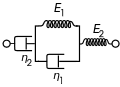# RC circuit help

• Engineering
Hello,

I have a question about RC circuit. Given this circuit

---R2---
---- R1---C1--| |----
----C2--

which is a resistor followed by a capacitor followed by a resistor in parallel with a capacitor.

I have trouble finding the derivative equation for the charging/decharging voltage and the corresponding current for the whole circuit. My goal is to have a derivative equation i can solve for each time step with a software I will create (this is not homework).

I am a programmer and I forgot my basics on electric circuits.

Help is very much appreciated

A.

berkeman
Mentor
Hello,

I have a question about RC circuit. Given this circuit

---R2---
---- R1---C1--| |----
----C2--

which is a resistor followed by a capacitor followed by a resistor in parallel with a capacitor.

I have trouble finding the derivative equation for the charging/decharging voltage and the corresponding current for the whole circuit. My goal is to have a derivative equation i can solve for each time step with a software I will create (this is not homework).

I am a programmer and I forgot my basics on electric circuits.

Help is very much appreciated

A.

Welcome to the PF.

It's a little hard to tell what the circuit is from the drawing so far. Is it a series RC in parallel with an R and a C?

How is the circuit driven? The drive circuit and its output impedance may need to be part of the solution.

In general, you would write the KCL equations for the circuit, using the differential equation relating voltage and current for the capacitor. Solving those equations would give you the characteristic equation for the circuit.

the circuit is as following:the kcl are as follows:

Utot=Un2+U(e1,n1)+Ue2
Itot=In2=Ie1 + In1=Ie2

the problem I am having is to find U(e1,n1), Un2, In1 and In2 relations because both capacitors affect the other and then I am always missing a variable.

Thanks.

A.

berkeman
Mentor

the circuit is as following:the kcl are as follows:

Utot=Un2+U(e1,n1)+Ue2
Itot=In2=Ie1 + In1=Ie2

the problem I am having is to find U(e1,n1), Un2, In1 and In2 relations because both capacitors affect the other and then I am always missing a variable.

Thanks.

A.

Those are not the KCL equations. And you need to show a driving source in order to have a full circuit.

I will post the full circuit with a source... but now I am confused, I had my last physics/electricity course 8 years ago and I thought kcl stands for kirchhoff circuit law which state that all incoming current should leave a node... therefore Itot=In2=Ie1 + In1=Ie2

I am kind of lost now

A.

berkeman
Mentor

I will post the full circuit with a source... but now I am confused, I had my last physics/electricity course 8 years ago and I thought kcl stands for kirchhoff circuit law which state that all incoming current should leave a node... therefore Itot=In2=Ie1 + In1=Ie2

I am kind of lost now

A.

That is the idea of the KCL, but that equation does not match the idea. The KCL for the left node in your drawing would be:

i(C2) + i(C1) + i(R1) = 0 (with appropriate signs on each term)

The current for C2 would be flowing to the left, and the current for C1 and R1 would be flowing to the right. You would write each current in terms of the voltage across each component. For the caps, you need to use the differential equation that relates current and voltage.

Here is the circuit:
[URL]http://people.ucalgary.ca/~widmera/circuit.jpg[/URL]

the circuit has a source of 5V

when charging:

C1 is the capacitor after the switch
R1 is the resistor after C1
R2 is the resistor in parallel
C2 is the capacitor in parallel

at the middle node between both resistor the KCL would look like this

I(R1)-I(R2)-I(C2)=0

where
I(R1)=I(C1)=v/R1+C1*dv(t)/dt
I(R2)=(v-v(R1)-v(C1))/R2
I(C2)=C2*d(v-v(R1)-v(C1))/dt

Am I going somewhere with this?
What should I do if I would like to know the step response of the whole circuit?

Last edited by a moderator:
Here is the circuit:
[URL]http://people.ucalgary.ca/~widmera/circuit.jpg[/URL]

the circuit has a source of 5V

when charging:

C1 is the capacitor after the switch
R1 is the resistor after C1
R2 is the resistor in parallel
C2 is the capacitor in parallel

at the middle node between both resistor the KCL would look like this

I(R1)-I(R2)-I(C2)=0

where
I(R1)=I(C1)=v/R1+C1*dv(t)/dt
I(R2)=(v-v(R1)-v(C1))/R2
I(C2)=C2*d(v-v(R1)-v(C1))/dt

Am I going somewhere with this?
What should I do if I would like to know the step response of the whole circuit?

Step response is the system response(output) to a unit step excitation(input). It's not clear where the input and output are.

Last edited by a moderator:
Lets say I would like to apply a constant 5V on the system as input and I would like to follow step by step the circuit charging...

I would like to know what the voltage in the circuit is at each time step v(t)

I have some progress from I(R1)-I(R2)-I(C2)=0

V(C1)=1/C1*integrate(I,dt)
V(R1)=R1*I
V(C2)=1/C2*integrate(I-I(R2),dt)=V(R2)=(I-I(C2))*R2

I(R1)=I(C1)=C1*dV(t)/dt=V/R1
I(C2)=C2*d(V-V(R1)-V(C1))/dt
I(R2)=(V-V(R1)-V(C1))/R2

Vtot=V(C1)+V(R1)+V(C2)=V(C1)+V(R1)+V(R2)

Itot=I(C1)=I(R1)=I(R2)+I(C2)

If I expand vtot:
Vtot=1/C1*integrate(I,dt)+R*I+(??) I have problem with the parallel circuit

Last edited:
If I understand well.. C2 will be charged rapidly (how fast??)... therefore after a short time the voltage drop will be equal to V=R2*Itot because no current goes through C2 anymore... if I follow this lead, this means that the capacitor holds a voltage equal to R2*Itot. So C1 will hold V(source)-V(R1)-R2*Itot when t=infinity

Does this make sense?

Last edited: